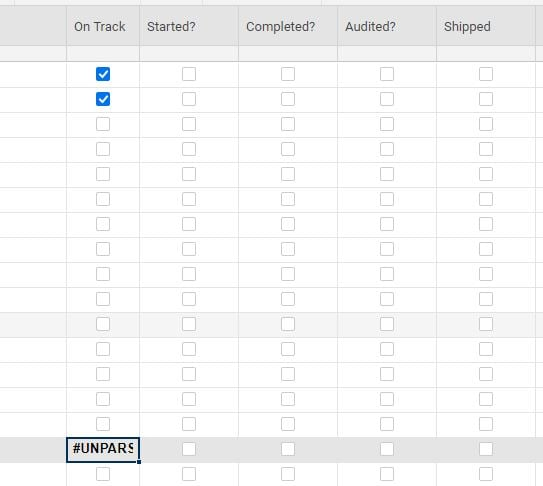# Formula for a checkbox column

I have searched all the forums for the formula for the checkbox sum & none of them have worked for me. I need to total the sum of the checkboxes that are checked within a column.Tags:

• I just needed to do this today.

enter '=COUNTIF', then select the entire column, and put '1' for checked.

=COUNTIF({On Track}, 1)

• I don't know why, but its not working for me.  I've tried this several times. I either get "unparseable" or Boolean Exp message.

• It worked if it is not in the same column as the checkboxes! Is there a way to make it part of the same column so the totals are below the boxes?

• If you make all of the lower rows Child rows (indent them) below the summary row, you could use...

=COUNTIFS(CHILDREN(), 1) + ""

thinkspi.com

• @Paul Newcome this formula helped me tremendously! Now I am facing another challenging. I am now trying to SUM the COUNTIFS totals using a CHILDREN formula to show the overarching total. I have attached an image. When I just try a general =SUM(CHILDREN( formula, nothing populates in the cell and I still see the checkbox. When I tried totaling manually at one point, instead of a SUM I somehow merged the numbers into a string (i.e. instead of 5 + 4 + 1 creating 10, I got 541).

Any suggestions on how I can total up the 23 + 17 + 1 + 0 + 0 + 0 appropriately in the North America row?

• @Jen V I am at a similar situation. Did you find a way to sum in the checkbox column?